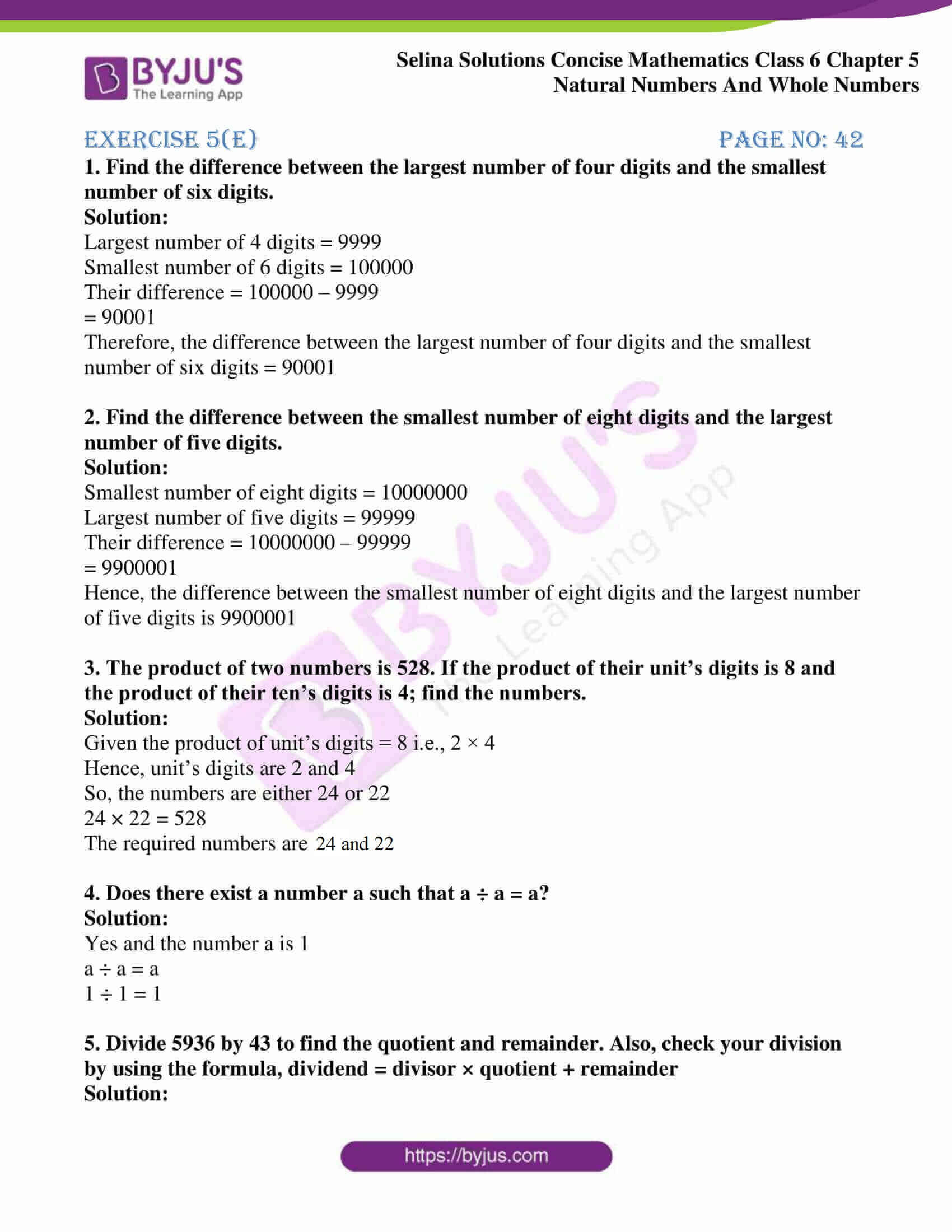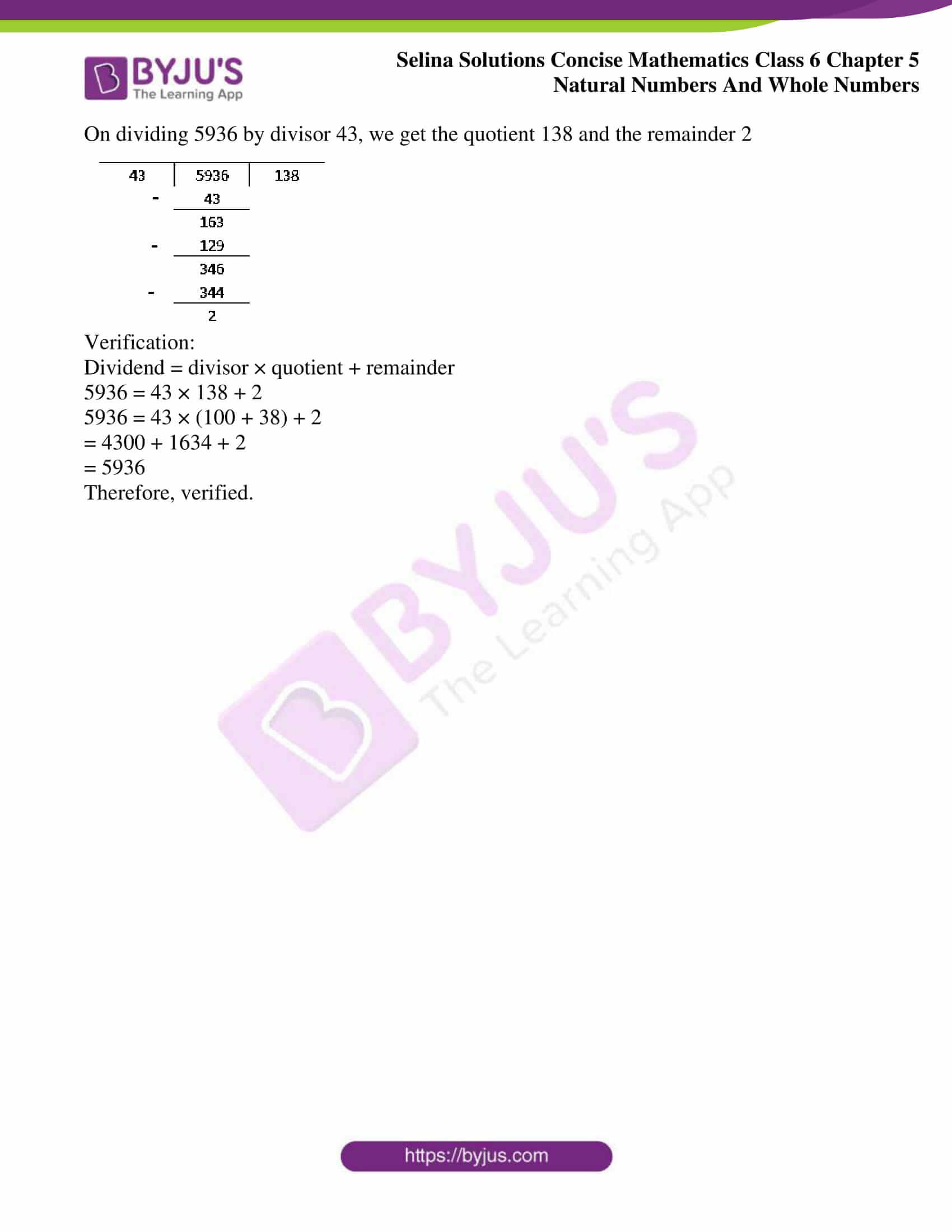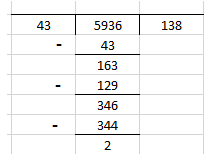# Selina Solutions Concise Mathematics Class 6 Chapter 5 Natural And Whole Numbers Exercise 5(E)

Selina Solutions Concise Mathematics Class 6 Chapter 5 Natural Numbers And Whole Numbers gives a clear idea of solving the textbook questions correctly. Mathematics is a subject that needs diligent practice by students to understand the concepts covered in-depth. Students who aspire to solve the questions confidently in the examination are suggested to refer to Selina Solutions created by experts at BYJU’S. This also boosts their performance in solving tricky questions of the textbook in a short duration. Students can use the PDF of Selina Solutions Concise Mathematics Class 6 Chapter 5 Natural Numbers And Whole Numbers Exercise 5(E), from the links mentioned below

## Selina Solutions Concise Mathematics Class 6 Chapter 5: Natural Numbers And Whole Numbers Exercise 5(E) Download PDF### Access other exercises of Selina Solutions Concise Mathematics Class 6 Chapter 5: Natural Numbers And Whole Numbers

Exercise 5(A) Solutions

Exercise 5(B) Solutions

Exercise 5(C) Solutions

Exercise 5(D) Solutions

Exercise 5(F) Solutions

### Access Selina Solutions Concise Mathematics Class 6 Chapter 5: Natural Numbers And Whole Numbers Exercise 5(E)

#### Exercise 5(E) page no: 42

1. Find the difference between the largest number of four digits and the smallest number of six digits.

Solution:

Largest number of 4 digits = 9999

Smallest number of 6 digits = 100000

Their difference = 100000 – 9999

= 90001

Therefore, the difference between the largest number of four digits and the smallest number of six digits = 90001

2. Find the difference between the smallest number of eight digits and the largest number of five digits.

Solution:

Smallest number of eight digits = 10000000

Largest number of five digits = 99999

Their difference = 10000000 – 99999

= 9900001

Hence, the difference between the smallest number of eight digits and the largest number of five digits is 9900001

3. The product of two numbers is 528. If the product of their unit’s digits is 8 and the product of their ten’s digits is 4; find the numbers.

Solution:

Given the product of unit’s digits = 8 i.e., 2 × 4

Hence, unit’s digits are 2 and 4

So, the numbers are either 24 or 22

24 × 22 = 528

The required numbers are 24 and 22

4. Does there exist a number a such that a ÷ a = a?

Solution:

Yes and the number a is 1

a ÷ a = a

1 ÷ 1 = 1

5. Divide 5936 by 43 to find the quotient and remainder. Also, check your division by using the formula, dividend = divisor × quotient + remainder

Solution:

On dividing 5936 by divisor 43, we get the quotient 138 and the remainder 2Verification:

Dividend = divisor × quotient + remainder

5936 = 43 × 138 + 2

5936 = 43 × (100 + 38) + 2

= 4300 + 1634 + 2

= 5936

Therefore, verified.